Скачать презентацию Abduction CIS 308 Dr Harry Erwin Three

5d25ca922c47382f63f2932a52735c44.ppt

• Количество слайдов: 21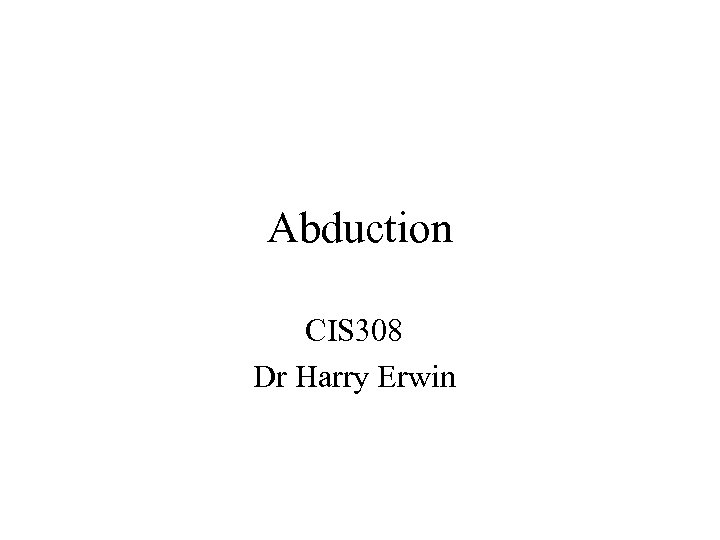Abduction CIS 308 Dr Harry Erwin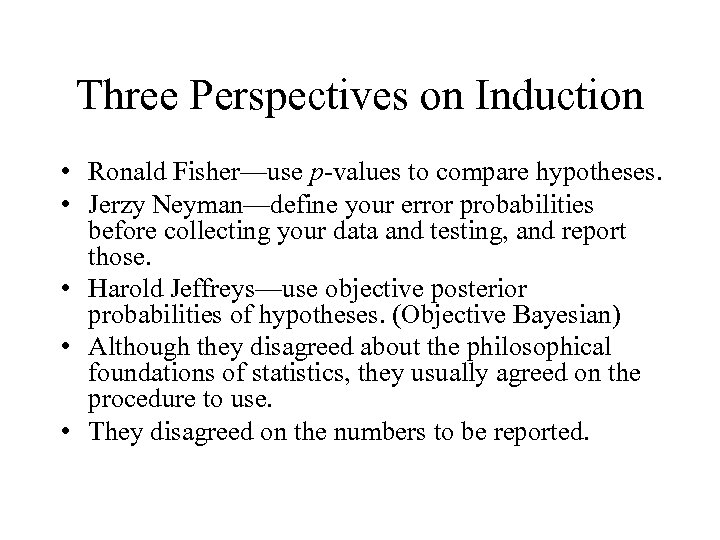Three Perspectives on Induction • Ronald Fisher—use p-values to compare hypotheses. • Jerzy Neyman—define your error probabilities before collecting your data and testing, and report those. • Harold Jeffreys—use objective posterior probabilities of hypotheses. (Objective Bayesian) • Although they disagreed about the philosophical foundations of statistics, they usually agreed on the procedure to use. • They disagreed on the numbers to be reported.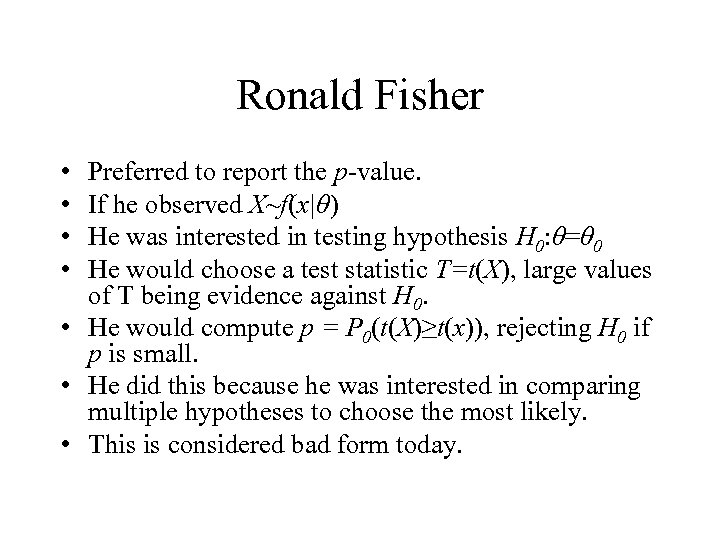Ronald Fisher • • Preferred to report the p-value. If he observed X~f(x|θ) He was interested in testing hypothesis H 0: θ=θ 0 He would choose a test statistic T=t(X), large values of T being evidence against H 0. • He would compute p = P 0(t(X)≥t(x)), rejecting H 0 if p is small. • He did this because he was interested in comparing multiple hypotheses to choose the most likely. • This is considered bad form today.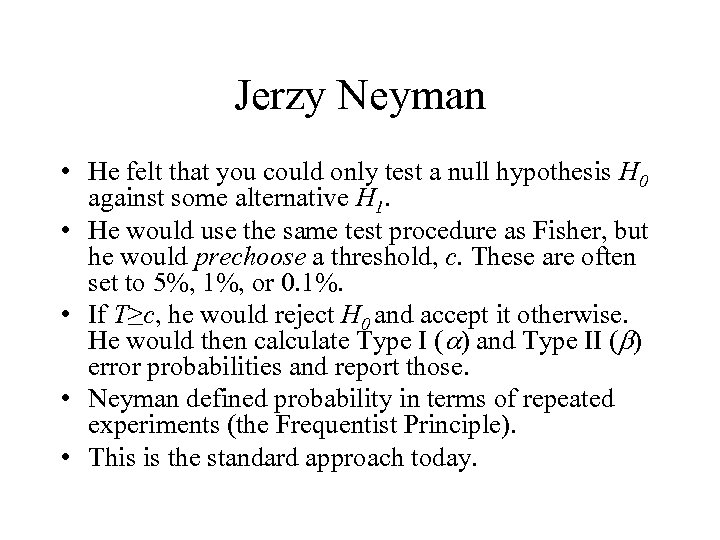Jerzy Neyman • He felt that you could only test a null hypothesis H 0 against some alternative H 1. • He would use the same test procedure as Fisher, but he would prechoose a threshold, c. These are often set to 5%, 1%, or 0. 1%. • If T≥c, he would reject H 0 and accept it otherwise. He would then calculate Type I (a) and Type II (b) error probabilities and report those. • Neyman defined probability in terms of repeated experiments (the Frequentist Principle). • This is the standard approach today.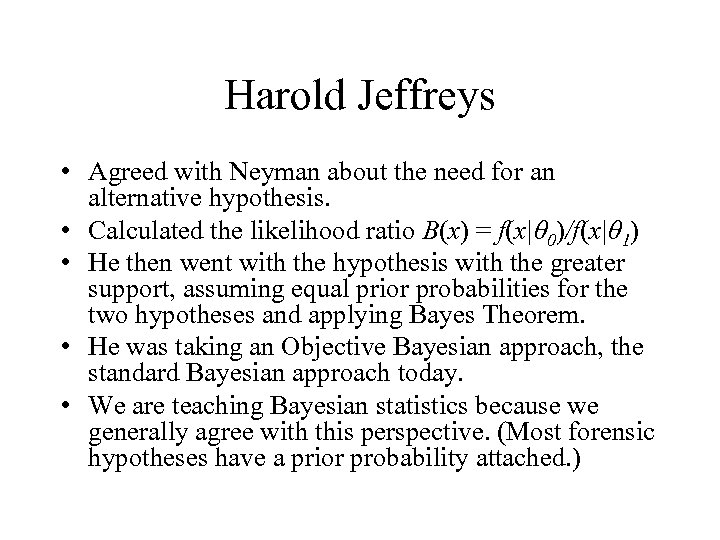Harold Jeffreys • Agreed with Neyman about the need for an alternative hypothesis. • Calculated the likelihood ratio B(x) = f(x|θ 0)/f(x|θ 1) • He then went with the hypothesis with the greater support, assuming equal prior probabilities for the two hypotheses and applying Bayes Theorem. • He was taking an Objective Bayesian approach, the standard Bayesian approach today. • We are teaching Bayesian statistics because we generally agree with this perspective. (Most forensic hypotheses have a prior probability attached. )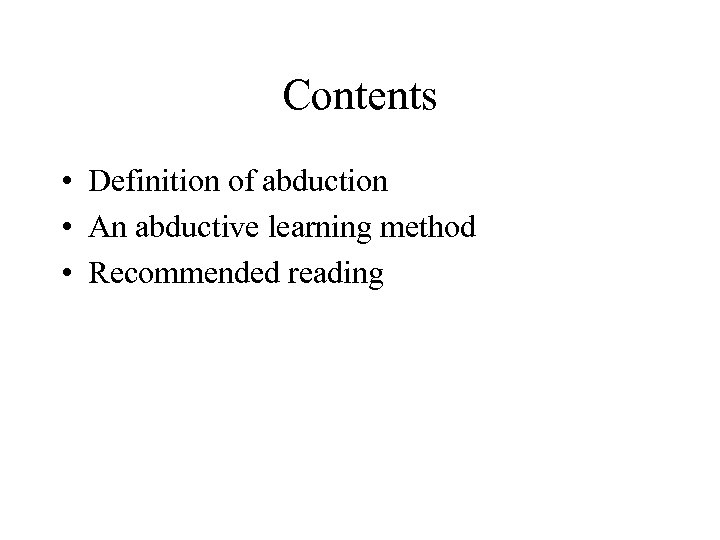Contents • Definition of abduction • An abductive learning method • Recommended reading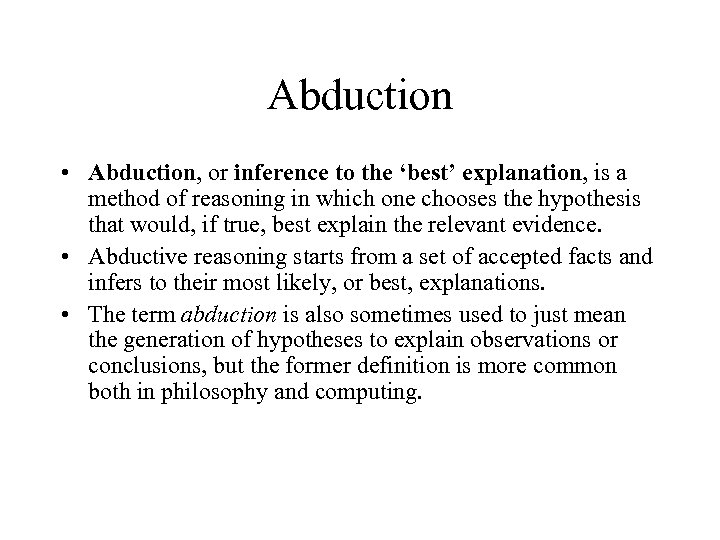Abduction • Abduction, or inference to the ‘best’ explanation, is a method of reasoning in which one chooses the hypothesis that would, if true, best explain the relevant evidence. • Abductive reasoning starts from a set of accepted facts and infers to their most likely, or best, explanations. • The term abduction is also sometimes used to just mean the generation of hypotheses to explain observations or conclusions, but the former definition is more common both in philosophy and computing.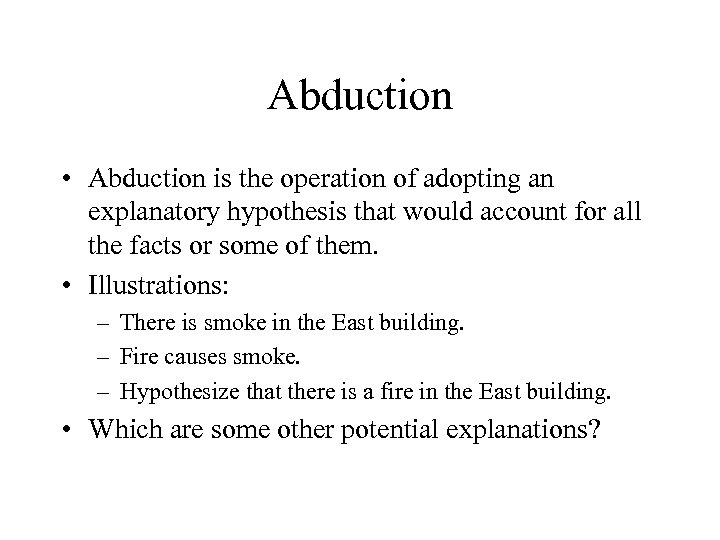Abduction • Abduction is the operation of adopting an explanatory hypothesis that would account for all the facts or some of them. • Illustrations: – There is smoke in the East building. – Fire causes smoke. – Hypothesize that there is a fire in the East building. • Which are some other potential explanations?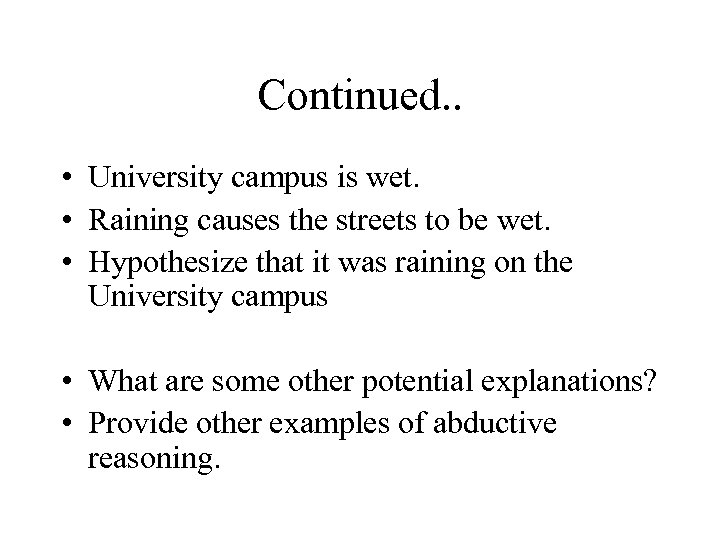Continued. . • University campus is wet. • Raining causes the streets to be wet. • Hypothesize that it was raining on the University campus • What are some other potential explanations? • Provide other examples of abductive reasoning.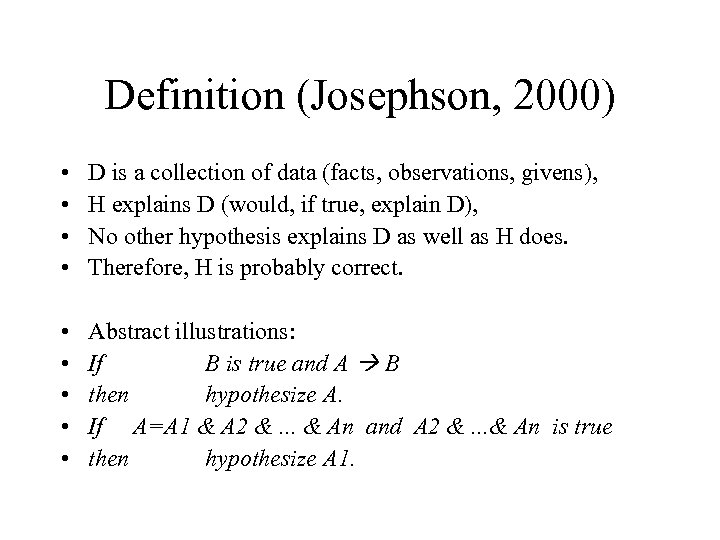Definition (Josephson, 2000) • • D is a collection of data (facts, observations, givens), H explains D (would, if true, explain D), No other hypothesis explains D as well as H does. Therefore, H is probably correct. • • • Abstract illustrations: If B is true and A B then hypothesize A. If A=A 1 & A 2 &. . . & An and A 2 &. . . & An is true then hypothesize A 1.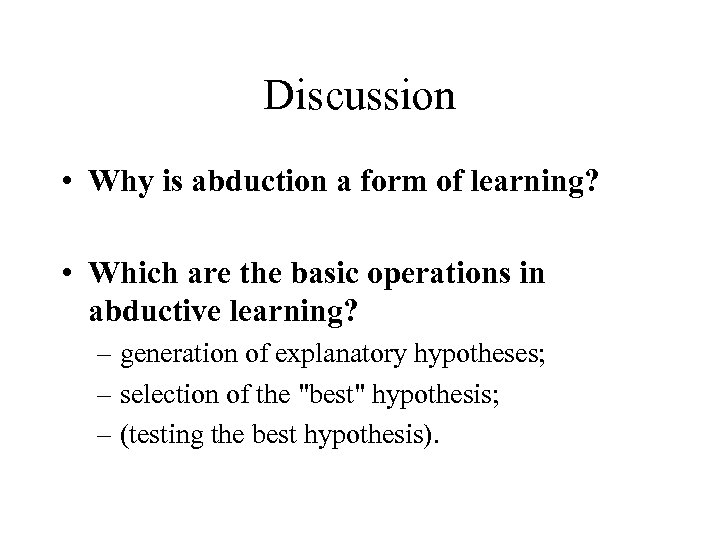Discussion • Why is abduction a form of learning? • Which are the basic operations in abductive learning? – generation of explanatory hypotheses; – selection of the "best" hypothesis; – (testing the best hypothesis).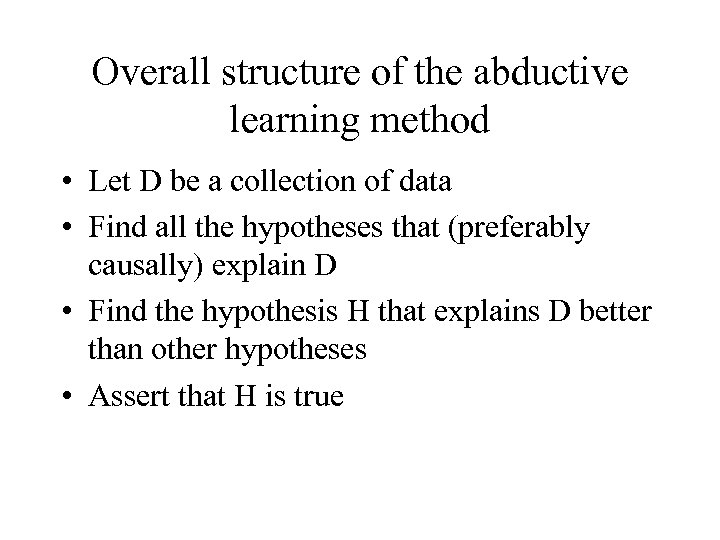Overall structure of the abductive learning method • Let D be a collection of data • Find all the hypotheses that (preferably causally) explain D • Find the hypothesis H that explains D better than other hypotheses • Assert that H is true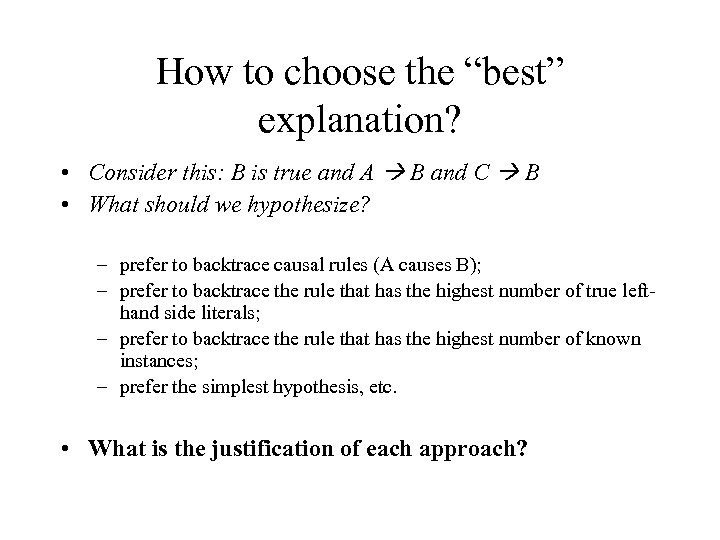How to choose the “best” explanation? • Consider this: B is true and A B and C B • What should we hypothesize? – prefer to backtrace causal rules (A causes B); – prefer to backtrace the rule that has the highest number of true lefthand side literals; – prefer to backtrace the rule that has the highest number of known instances; – prefer the simplest hypothesis, etc. • What is the justification of each approach?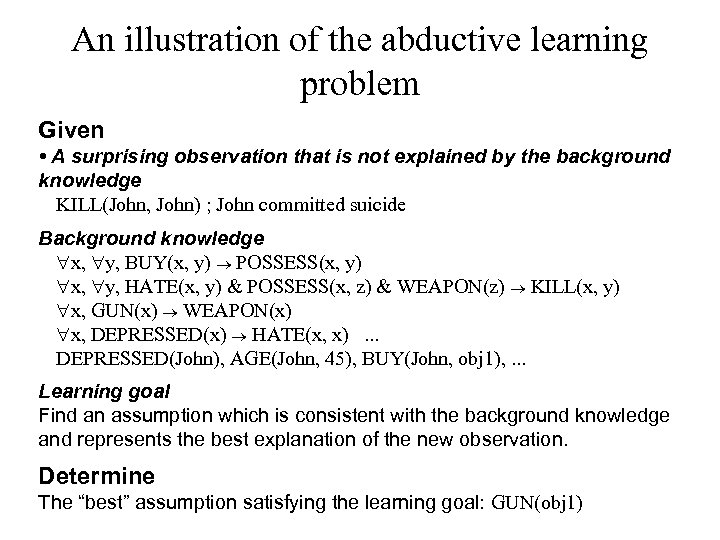An illustration of the abductive learning problem Given • A surprising observation that is not explained by the background knowledge KILL(John, John) ; John committed suicide Background knowledge "x, "y, BUY(x, y) ® POSSESS(x, y) "x, "y, HATE(x, y) & POSSESS(x, z) & WEAPON(z) ® KILL(x, y) "x, GUN(x) ® WEAPON(x) "x, DEPRESSED(x) ® HATE(x, x). . . DEPRESSED(John), AGE(John, 45), BUY(John, obj 1), . . . Learning goal Find an assumption which is consistent with the background knowledge and represents the best explanation of the new observation. Determine The “best” assumption satisfying the learning goal: GUN(obj 1)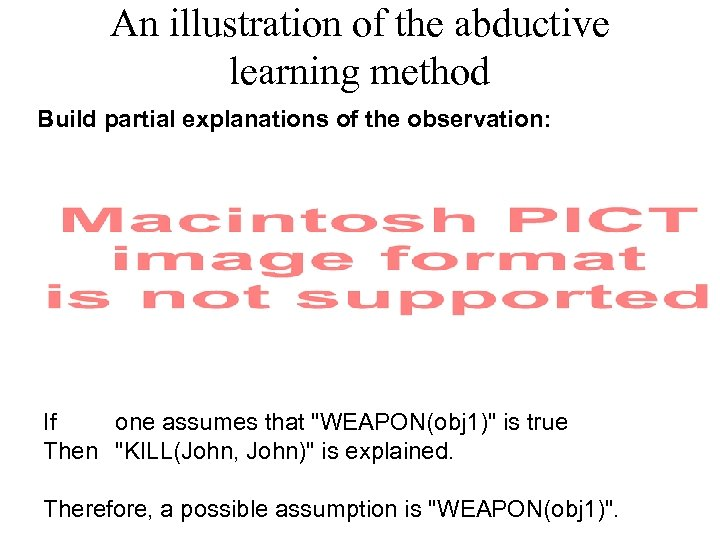An illustration of the abductive learning method Build partial explanations of the observation: If one assumes that "WEAPON(obj 1)" is true Then "KILL(John, John)" is explained. Therefore, a possible assumption is "WEAPON(obj 1)".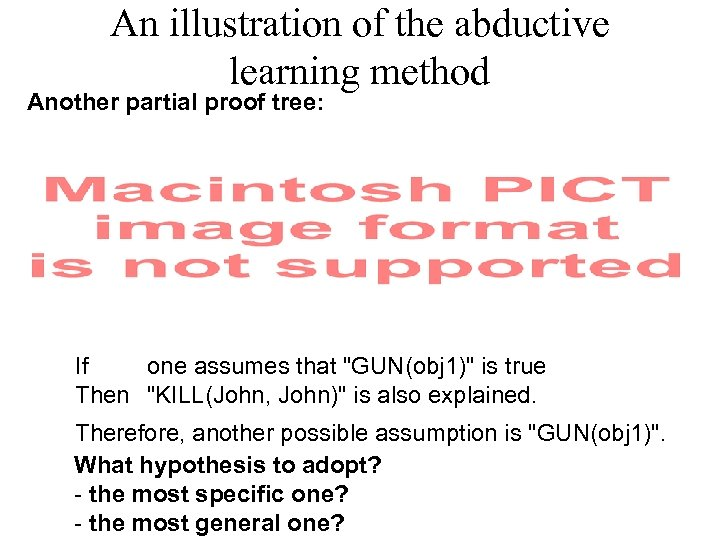An illustration of the abductive learning method Another partial proof tree: If one assumes that "GUN(obj 1)" is true Then "KILL(John, John)" is also explained. Therefore, another possible assumption is "GUN(obj 1)". What hypothesis to adopt? - the most specific one? - the most general one?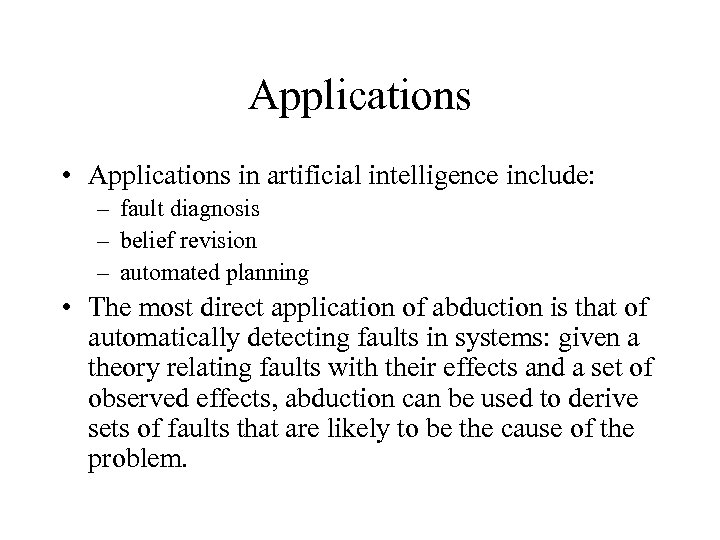Applications • Applications in artificial intelligence include: – fault diagnosis – belief revision – automated planning • The most direct application of abduction is that of automatically detecting faults in systems: given a theory relating faults with their effects and a set of observed effects, abduction can be used to derive sets of faults that are likely to be the cause of the problem.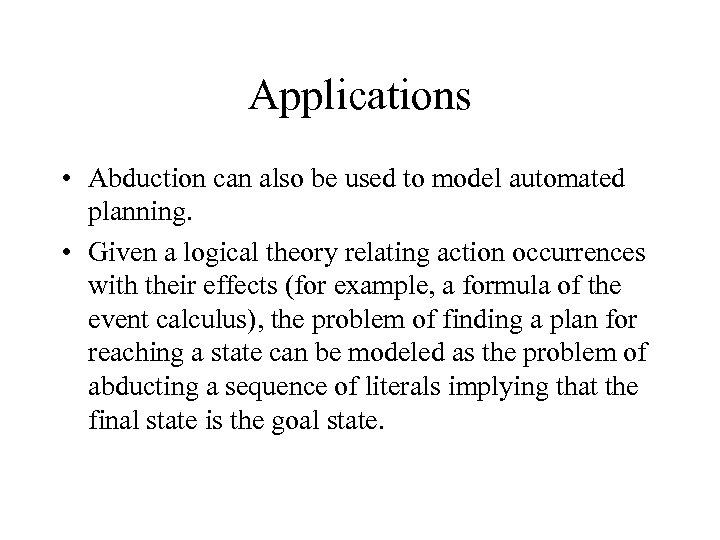Applications • Abduction can also be used to model automated planning. • Given a logical theory relating action occurrences with their effects (for example, a formula of the event calculus), the problem of finding a plan for reaching a state can be modeled as the problem of abducting a sequence of literals implying that the final state is the goal state.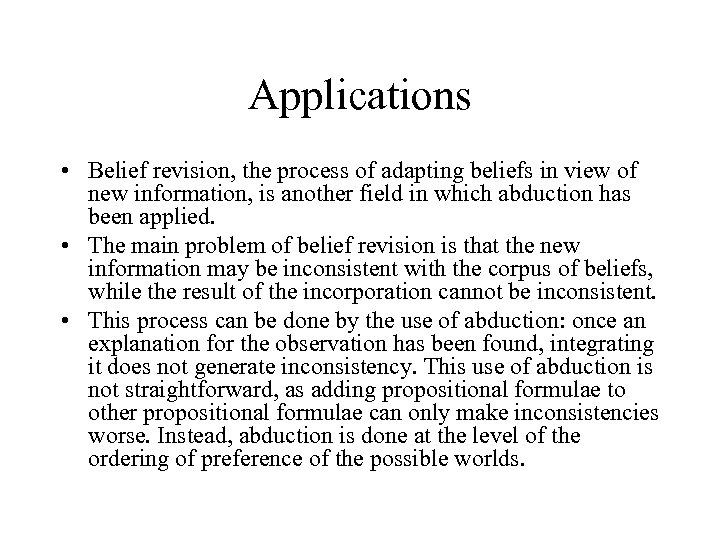Applications • Belief revision, the process of adapting beliefs in view of new information, is another field in which abduction has been applied. • The main problem of belief revision is that the new information may be inconsistent with the corpus of beliefs, while the result of the incorporation cannot be inconsistent. • This process can be done by the use of abduction: once an explanation for the observation has been found, integrating it does not generate inconsistency. This use of abduction is not straightforward, as adding propositional formulae to other propositional formulae can only make inconsistencies worse. Instead, abduction is done at the level of the ordering of preference of the possible worlds.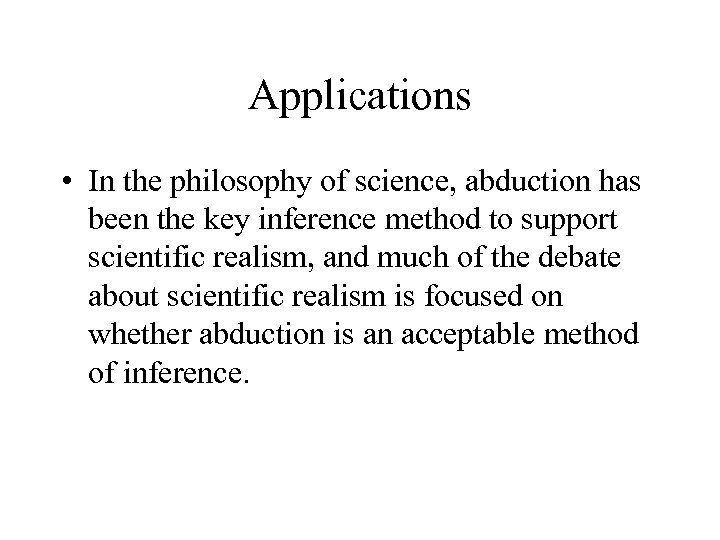Applications • In the philosophy of science, abduction has been the key inference method to support scientific realism, and much of the debate about scientific realism is focused on whether abduction is an acceptable method of inference.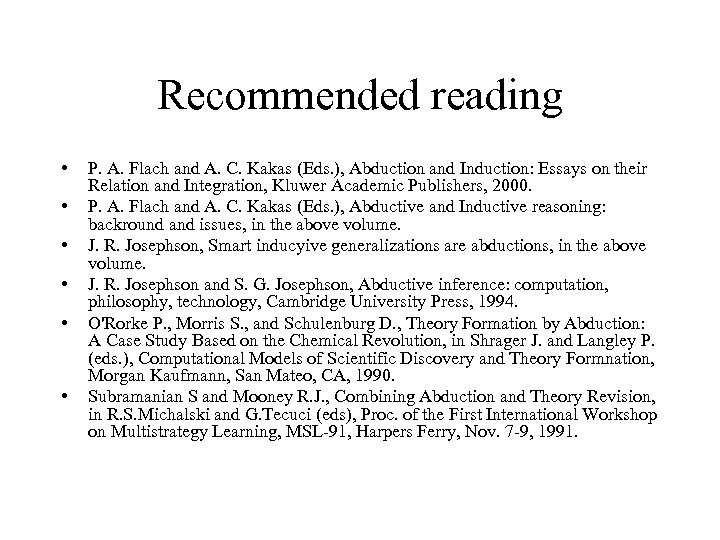Recommended reading • • • P. A. Flach and A. C. Kakas (Eds. ), Abduction and Induction: Essays on their Relation and Integration, Kluwer Academic Publishers, 2000. P. A. Flach and A. C. Kakas (Eds. ), Abductive and Inductive reasoning: backround and issues, in the above volume. J. R. Josephson, Smart inducyive generalizations are abductions, in the above volume. J. R. Josephson and S. G. Josephson, Abductive inference: computation, philosophy, technology, Cambridge University Press, 1994. O'Rorke P. , Morris S. , and Schulenburg D. , Theory Formation by Abduction: A Case Study Based on the Chemical Revolution, in Shrager J. and Langley P. (eds. ), Computational Models of Scientific Discovery and Theory Formnation, Morgan Kaufmann, San Mateo, CA, 1990. Subramanian S and Mooney R. J. , Combining Abduction and Theory Revision, in R. S. Michalski and G. Tecuci (eds), Proc. of the First International Workshop on Multistrategy Learning, MSL-91, Harpers Ferry, Nov. 7 -9, 1991.